# Data sufficiency Quiz for RRB PO & RRB CLERK : Quiz – 9

## Data sufficiency Quiz for RRB PO & RRB CLERK : Quiz – 9

Data Sufficiency Questions appear regularly in many competitive tests, so you can’t afford to ignore them. Many applicants find it difficult to answer Data Sufficiency Questions. If you practice enough Data Sufficiency Quiz questions, it will become a cakewalk for you. So here we bring to you Data Sufficiency Quiz to assist you in your preparation for upcoming exams like as banking, insurance, SSC, and railway. Aspirants can take this Data Sufficiency Quiz for free. This Data Sufficiency Quiz contains detailed explanation of each question. This Data Sufficiency Quiz is based on the most recent or updated exam pattern.

Directions (1 – 10): Each question below is followed by two or three statements A, B, and C. You have to determine whether the data given in the statements are sufficient for answering the question. You should use the data and your knowledge of Mathematics to choose between the possible answers. Read the statements and Answer.

1. Compound interest on a sum is Rs. 2100. Find the time for which it is lent?
(A) Difference between compound interest and Simple Interest on that sum for that time is Rs. 100.
(B) C.I. for this time on Rs. 5000 at 10% p.a. is equal to S.I. in 3 years on Rs. 3500 at same rate.
(C) If sum become half, rate and time is same then the sum amounts to Rs. 6050 at compound interest.
(a) Any two of them
(b) A and C together
(c) B and either A or C
(d) Either A or B
(e) Only B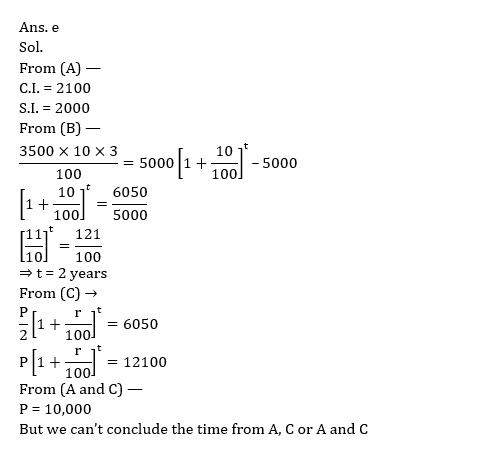2. What will be the length of the train A, if it crosses a train B of 120 m length coming from opposite direction?
(A) Relative speed of the train is 120 km/hr if they run in opposite directions
(B) Train A can cross a pole in 12 sec. whereas train B can cross a pole in 7.2 sec.
(C) Train A can cross a platform of 380 m length in 34.8 sec whereas train B can cross same platform in 30 sec.

(a) Any two of them
(b) Either B or C
(c) B and either A or C
(d) Either A or B
(e) Only B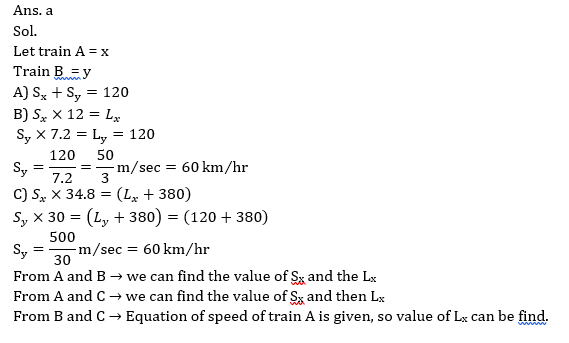3. In how many days 5 men and 3 women can complete the work?
(A) If 1 men and 1 women work on alternate days they can complete the work in 40 days.
(B) 15 men can complete the work in 4 days.
(C) Efficiency of men is half then that of women.
(a) A and either B or C
(b) Any one of them
(c) Any two of them
(d) Either A or B
(e) Either B or C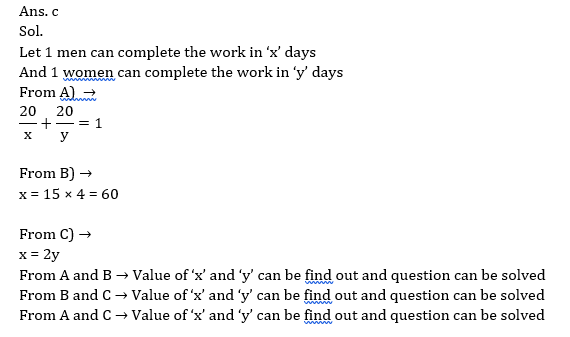4. An article is sold at 20% discount on MP. Find the gain percentage
(A) Marked price is 25% more than the selling price of the article.
(B) Cost price of the article is 40% less than the marked price of the article.
(C) Ratio of cost price and marked price is 3 : 5.
(a) A and either B or C
(b) Any one of them
(c) Any two of them
(d) Either A or B
(e) Either B or C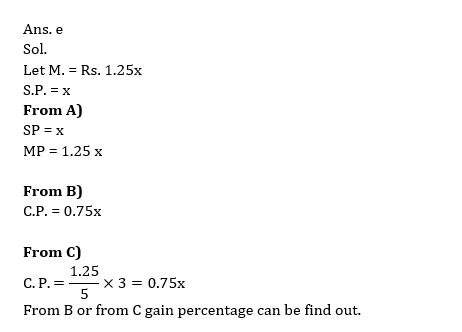5. What is the first term of an arithmetic progression?
A. Fifth term of the AP is 12 more than the third term of the AP.
B. The average of first three terms is 10.
C. The sum of fourth and sixth terms is 56.
(a) Only A and B together
(b) Only A and C together
(c) Only B and C together
(d) Any two of the three together
(e) All the three together6. What is the speed of the boat in still water?
A. The boat goes 80 km downstream in 4 hours.
B. Difference in the downstream and upstream speed is 8 km/h.
C. The boat goes 60 km upstream in 5 hours.
(a) Any two of the three together
(b) Only A and C together
(c) All the three together
(d) Only A and B together
(e) Only B and C together7. What is the area of a square?
A. The length of a diagonal of the square is 20 cm.
B. The length of an edge of the square is same as the length of a rectangle with area 120 cm2 and breadth cm.
C. Perimeter of the square is cm.
(a) Only A alone
(b) Only B alone
(c) Only C alone
(d) Either B alone or C alone
(e) Any one of the three alone8. There are some boys and some girls in a class. What is the probability of selecting a girl randomly from the students of the class?
A. Ratio of number of boys and girls in the class is 5 : 3.
B. The number of boys in the class is 20 more than of girls.
C. Total number of students in the class is 80.
(a) Either A alone or B and C together
(b) All the three together are not sufficient
(c) Only A alone
(d) Only B and C together
(e) All the three together9. What is the cost price of an article?
A. Shopkeeper has a profit of Rs.100 on selling the article.
B. The percentage mark up on the article is 25%.
C. The marked price of the article is Rs.750.
(a) Only A and B together
(b) All the three together
(c) Only B and C together
(d) Only A and C together
(e) Any two of three together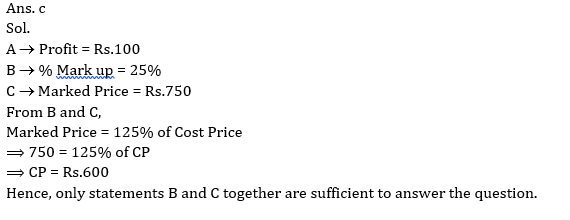#### Attempt Quantitative Aptitude Topic Wise Online Test Series

Recommended PDF’s for :

#### Most important PDF’s for Bank, SSC, Railway and Other Government Exam : Download PDF Now

AATMA-NIRBHAR Series- Static GK/Awareness Practice Ebook PDF Get PDF here
The Banking Awareness 500 MCQs E-book| Bilingual (Hindi + English) Get PDF here
AATMA-NIRBHAR Series- Banking Awareness Practice Ebook PDF Get PDF here
Computer Awareness Capsule 2.O Get PDF here
AATMA-NIRBHAR Series Quantitative Aptitude Topic-Wise PDF Get PDF here
AATMA-NIRBHAR Series Reasoning Topic-Wise PDF Get PDF Here
Memory Based Puzzle E-book | 2016-19 Exams Covered Get PDF here
Caselet Data Interpretation 200 Questions Get PDF here
Puzzle & Seating Arrangement E-Book for BANK PO MAINS (Vol-1) Get PDF here
ARITHMETIC DATA INTERPRETATION 2.O E-book Get PDF here
3